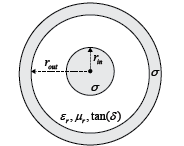# txlineCoaxial

Create coaxial transmission line

## Description

Use the `txlineCoaxial` object to create a coaxial transmission line. A cross-section of a coaxial transmission line is shown in this figure. The physical characteristics of a coaxial transmission line include the radius of the inner conductor, rin and the radius of the outer conductor, rout.## Creation

### Syntax

``coaxialtxline = txlineCoaxial``
``coaxialtxline = txlineCoaxial(Name,Value)``

### Description

````coaxialtxline = txlineCoaxial` creates a default coaxial transmission line object.```

example

````coaxialtxline = txlineCoaxial(Name,Value)` sets Properties using one or more name-value pairs. For example, ```txline = txlineCoaxial('OuterRadius',0.0046)``` creates a coaxial transmission line with an outer radius of `0.0046` meters.```

## Properties

expand all

Name of the coaxial transmission line, specified as a string scalar or a character vector.

Example: `'Name','coaxial1'`

Example: `coaxialtxline.Name = 'coaxial1'`

Data Types: `char` | `string`

Outer conductor radius, specified as a positive scalar in meters.

Example: `'OuterRadius',0.0074`

Example: `coaxialtxline.OuterRadius = 0.0074`

Data Types: `double`

Inner conductor radius, specified as a positive scalar in meters.

Example: `'InnerRadius',0.000875`

Example: `coaxialtxline.InnerRadius = 0.000875`

Data Types: `double`

Relative permeability of the dielectric, specified as a positive scalar.

Relative permeability is the ratio of the permeability of the dielectric, μ, to the permeability in free space, μ0.

Example: `'MuR',1.5`

Example: `coaxialtxline.MuR = 1.5`

Data Types: `double`

Relative permittivity of the dielectric, specified as a positive scalar.

Example: `'EpsilonR',1.4`

Example: `coaxialtxline.EpsilonR = 1.4`

Data Types: `double`

Loss angle tangent of the dielectric, specified as a nonnegative scalar.

Example: `'LossTangent',1`

Example: `coaxialtxline.LossTangent = 1`

Data Types: `double`

Conductivity of the conductor, specified as a nonnegative scalar in Siemens per meter (S/m).

Example: `'SigmaCond',2`

Example: `coaxialtxline.SigmaCond = 2`

Data Types: `double`

Physical length of the coaxial transmission line, specified as a positive scalar in meters.

Example: `'LineLength',0.2`

Example: `coaxialtxline.LineLength = 0.2`

Data Types: `double`

Stub transmission line termination, specified as `'NotApplicable'`, `'Open'`, or `'Short'`.

Example: `'Termination','Short'`

Example: `coaxialtxline.Termination = 'Short'`

Data Types: `char`

Type of stub, specified as `'NotAStub'`, `'Series'`, or `'Shunt'`.

Example: `'StubMode','Series'`

Example: `coaxialtxline.StubMode = 'Series'`

Data Types: `char`

This property is read-only.

Number of input and output ports, specified as a positive scalar.

Data Types: `double`

This property is read-only.

Terminals of coaxial transmission line, specified as cell array of strings.

Data Types: `char` | `string`

## Object Functions

 `sparameters` Calculate S-parameters for RF data, network, circuit, and matching network objects `groupdelay` Group delay of S-parameter object or RF filter object or RF Toolbox circuit object `noisefigure` Calculate noise figure of transmission lines, series RLC, and shunt RLC circuits `getZ0` Calculate characteristic impedance with and without dispersion for transmission line `circuit` Circuit object `clone` Create copy of existing circuit element or circuit object

## Examples

collapse all

Create a coaxial transmission line.

```txline = txlineCoaxial('OuterRadius',1.47e-3,'InnerRadius',0.45e-3,... 'EpsilonR',3.4,'SigmaCond',5.8e7);```

Calculate the characteristic impedance with dispersion for the coaxial transmission line at at the frequency of `6` GHz.

`z0_f = getZ0(txline,6e9)`
```z0_f = 38.4927 - 0.0201i ```

## Version History

Introduced in R2020b